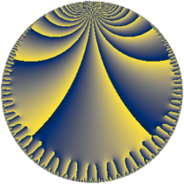# Properties

 Label 95.11.mLevel $95$ Weight $11$ Character orbit 95.m Rep. character $\chi_{95}(7,\cdot)$ Character field $\Q(\zeta_{12})$ Dimension $392$ Newform subspaces $1$ Sturm bound $110$ Trace bound $0$

# Related objects

## Defining parameters

 Level: $$N$$ $$=$$ $$95 = 5 \cdot 19$$ Weight: $$k$$ $$=$$ $$11$$ Character orbit: $$[\chi]$$ $$=$$ 95.m (of order $$12$$ and degree $$4$$) Character conductor: $$\operatorname{cond}(\chi)$$ $$=$$ $$95$$ Character field: $$\Q(\zeta_{12})$$ Newform subspaces: $$1$$ Sturm bound: $$110$$ Trace bound: $$0$$

## Dimensions

The following table gives the dimensions of various subspaces of $$M_{11}(95, [\chi])$$.

Total New Old
Modular forms 408 408 0
Cusp forms 392 392 0
Eisenstein series 16 16 0

## Trace form

 $$392 q - 2 q^{2} - 2 q^{3} + 2220 q^{5} + 4856 q^{6} + 7780 q^{7} - 142356 q^{8} + O(q^{10})$$ $$392 q - 2 q^{2} - 2 q^{3} + 2220 q^{5} + 4856 q^{6} + 7780 q^{7} - 142356 q^{8} - 2 q^{10} + 32064 q^{11} - 232108 q^{12} - 818490 q^{13} - 1141388 q^{15} + 49026272 q^{16} + 2072126 q^{17} + 4464336 q^{18} - 30123952 q^{20} - 1448284 q^{21} + 4905706 q^{22} + 131242 q^{23} - 5318128 q^{25} + 35863504 q^{26} - 40337048 q^{27} + 35162792 q^{28} - 626212 q^{30} - 64672896 q^{31} + 101446340 q^{32} + 16944736 q^{33} + 97780658 q^{35} - 1696907108 q^{36} + 188603000 q^{37} + 316760754 q^{38} + 440672472 q^{40} - 428613964 q^{41} - 774149798 q^{42} - 203275548 q^{43} + 445246440 q^{45} - 1583809336 q^{46} + 827682184 q^{47} + 93769212 q^{48} + 3444668164 q^{50} - 375042204 q^{51} - 1680459778 q^{52} - 312762144 q^{53} - 922476300 q^{55} + 1245970416 q^{56} - 1399302072 q^{57} + 5178550404 q^{58} + 2790379518 q^{60} + 2729471636 q^{61} + 1463639316 q^{62} - 5716734310 q^{63} - 5741012704 q^{65} - 14278935268 q^{66} - 1123450394 q^{67} + 2843511172 q^{68} + 5385951110 q^{70} + 3202662556 q^{71} - 10998992704 q^{72} + 4692302722 q^{73} + 35410377548 q^{75} - 4934546772 q^{76} + 13472322668 q^{77} + 3477052916 q^{78} - 3697722930 q^{80} + 61033508952 q^{81} - 14484700660 q^{82} + 6779152124 q^{83} - 20028400922 q^{85} + 24296217656 q^{86} - 17074613364 q^{87} + 9785438184 q^{88} - 10206419488 q^{90} + 46291684236 q^{91} - 17646129964 q^{92} - 14290061476 q^{93} - 9651375010 q^{95} - 147008832576 q^{96} - 12701510184 q^{97} - 535132524 q^{98} + O(q^{100})$$

## Decomposition of $$S_{11}^{\mathrm{new}}(95, [\chi])$$ into newform subspaces

Label Dim $A$ Field CM Traces $q$-expansion
$a_{2}$ $a_{3}$ $a_{5}$ $a_{7}$
95.11.m.a $392$ $60.359$ None $$-2$$ $$-2$$ $$2220$$ $$7780$$